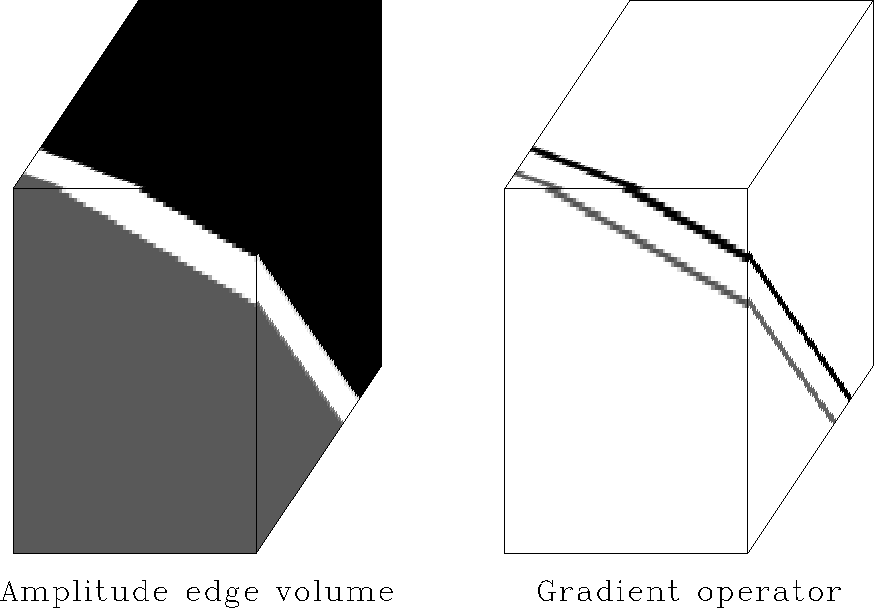Next: Results Up: Standard edge detection Previous: Standard edge detection

In Figure 13, a gradient magnitude operator detects the amplitude edges at which pixels change their gray-level suddenly. For an image volume,the magnitude of the gradient vector(1)
assumes a local maximum at an amplitude edge. The magnitude is zero, if f is constant.Figure 13
Gradient magnitude operator applied to constant amplitude image. The input image on the left consists of three regions of constant amplitude. The output of the gradient magnitude operator on the right delineates the edges: two parallel plane surfaces. The pixel amplitudes are all non-negative. Large amplitudes in the output image indicate edges in the input image. Zero output amplitude indicates locally constant input.

In the case of digital images, partial derivatives are conveniently computed by finite-difference approximations. In particular, I use a three-dimensional generalization of(2)
to approximate the horizontal partial derivative of this chapter's image volumes. Table 2 lists alternative finite-difference approximations of the partial derivative operator. The various masks vary in their moments, which affects the operators' isotropy, robustness and resolution. Averaging of surrounding values before subtraction reduces the effect of noise and increases the sensitivity to gradual grey-level changes. However, an increase in operator size causes a decrease in the operator's ability to resolve two neighboring edges. The finite-difference approximations illustrate the prediction character of the filter: the difference between the two neighboring pixels is the error one makes predicting one amplitude value to be the same as the next.

 Roberts Smoothed Prewitt Expanded Prewitt Sobel Isotropic () 1 -1 0 $1 -1 1 -1 1$ -1 -1 -1 1 1 -1 -1 1 1 -1 -1 1 1 -1 -1 1 1 $-2 2 -1 1$ -2 2 -1 1 \$

The Derivative Theorem of the Fourier Transform,

shows that the partial derivative operator scales each Fourier component of the input image by its spatial wavenumber in the derivative direction. Consequently, the high frequency components that constitute an edge are amplified and low frequency components that constitute near constant amplitude areas are suppressed. The zero frequency component is erased. Additionally, the derivative operator causes a 900 degree phase shift.Next: Results Up: Standard edge detection Previous: Standard edge detection
Stanford Exploration Project
3/8/1999